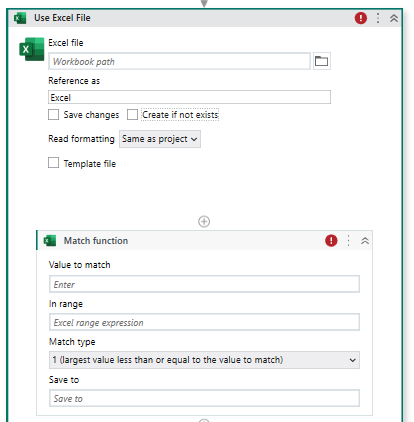# Compare one by one cell value from 2 sheet

Hello,

I have 2 sheets that need compare 1by1. if any cell is not equal will stop.

If 1 = 1’ (row1: 101.64.86050) true → 2=2’ → 3=3’ → 4=4’
1 = 1’ (row2: 114.35.36450) true → 2=2’ → 3=3’ → 4=4’

Check “OK” or “NO” at I(Result sheet)

I can get & compare values at 1& 1’ but another cell can’t get, always not equal. Thank all
DLV030_2.xaml (23.0 KB)
DLV030.xls (31 KB)

Hi @anh.nguyen

1. Use the “Excel Application Scope” activity to open your first sheet.
2. Use the “Read Range” activity to read the data from your first sheet into a DataTable variable.
3. Use the “Excel Application Scope” activity again to open your second sheet.
4. Use the “Read Range” activity to read the data from your second sheet into a second DataTable variable.
5. Use a “For Each Row” activity to loop through each row in the first DataTable.
6. Inside the “For Each Row” activity, use an “If” activity to compare the cells in each row of the two DataTables.
7. If any cell is not equal, set a boolean variable (e.g. “match”) to false and exit the “For Each Row” loop.
8. If all cells match, set the “match” variable to true.
9. Use the “Append Range” activity to write “OK” or “NO” to the result sheet, based on the value of the “match” variable.

Regards,
Kaviyarasu N

1 Like

In other hand use read range actions for read the two sheet value.

Use match function inside for each datatable actions